# CS 109A/AC 209A/STAT 121A Data Science: Homework 5¶

Harvard University
Fall 2016
Instructors: W. Pan, P. Protopapas, K. Rader
Due Date: Wednesday, October 26th, 2016 at 11:59pm

To submit your assignment, in Vocareum, upload (using the 'Upload' button on your Vocareum Jupyter Dashboard) your solution to Vocareum as a single notebook with following file name format:

last_first_CourseNumber_HW5.ipynb

where CourseNumber is the course in which you're enrolled (CS 109a, Stats 121a, AC 209a). Submit your assignment in Vocareum using the 'Submit' button.

Avoid editing your file in Vocareum after uploading. If you need to make a change in a solution. Delete your old solution file from Vocareum and upload a new solution. Click submit only ONCE after verifying that you have uploaded the correct file. The assignment will CLOSE after you click the submit button.

Problems on homework assignments are equally weighted. The Challenge Question is required for AC 209A students and optional for all others. Student who complete the Challenge Problem as optional extra credit will receive +0.5% towards your final grade for each correct solution.

In :
# Clear namespace
for name in dir():
if not name.startswith('_'):
del globals()[name]


Import libraries

In :
# Data manipulation
import numpy as np
import pandas as pd
import re

# Ploting
import matplotlib
import matplotlib.pyplot as plt
import matplotlib.mlab as mlab
from mpl_toolkits.mplot3d import Axes3D
import matplotlib.cm as cmx
import matplotlib.colors as colors

# Statistics
import statsmodels.api as sm
from scipy.stats import norm
from collections import Counter

# Machine Learning
from sklearn import linear_model
from sklearn.cross_validation import train_test_split
from sklearn.feature_extraction.text import CountVectorizer
from sklearn.neighbors import KNeighborsClassifier as KNN
from sklearn.linear_model import LogisticRegressionCV as LogRegCV
from sklearn.linear_model import LogisticRegression as LogReg
from sklearn.decomposition import PCA
from sklearn.preprocessing import PolynomialFeatures
from sklearn.feature_extraction.text import TfidfTransformer
from sklearn.metrics import confusion_matrix
import nltk
%matplotlib inline


## Problem 0: Basic Information¶

[Hagmann, Tim]

[CS 109a]

### Part (c): Who did you work with?¶

[-]

All data sets can be found in the datasets folder and are in comma separated value (CSV) format

## Problem 1: Image Classification¶

In this problem, your task is to classify images of handwritten digits.

The data set is provided in the file dataset_1.txt and contains 8x8 gray-scale images of hand-written digits, flattened to a 64-length vector. The last column contains the digit. For simplicity, we have only included digits 0, 1 and 3.

We want you to build a model that can be given the image of a hand-written digit and correctly classify this digit as 0, 1 or 3.

### Step 1: Read in the data and visualize¶

In :
#Load the data

#Split into predictor and response
x = data[:, :-1]
y = data[:, -1]

#Print shapes of predictor and response arrays
print 'predictor matrix shape:', x.shape
print 'response array shape:', y.shape

predictor matrix shape: (543L, 64L)
response array shape: (543L,)


## Visualise the data:¶

In :
#Plot a couple of images from the dataset
fig, ax = plt.subplots(2, 3, figsize=(15, 5))

#Plot the 0th image vector
ax[0, 0].imshow(x.reshape(8, 8), cmap=plt.cm.gray_r)
ax[0, 0].set_title('0-th image vector')

#Plot the 300th image vector
ax[0, 1].imshow(x.reshape(8, 8), cmap=plt.cm.gray_r)
ax[0, 1].set_title('300-th image vector')

#Plot the 400th image vector
ax[0, 2].imshow(x.reshape(8, 8), cmap=plt.cm.gray_r)
ax[0, 2].set_title('400-th image vector')

#Plot the 0th image vector, with de-blurring
ax[1, 0].imshow(x.reshape(8, 8), cmap=plt.cm.gray_r, interpolation='nearest')
ax[1, 0].set_title('0-th image vector, de-blurred')

#Plot the 300th image vector, with de-blurring
ax[1, 1].imshow(x.reshape(8, 8), cmap=plt.cm.gray_r, interpolation='nearest')
ax[1, 1].set_title('300-th image vector, de-blurred')

#Plot the 400th image vector, with de-blurring
ax[1, 2].imshow(x.reshape(8, 8), cmap=plt.cm.gray_r, interpolation='nearest')
ax[1, 2].set_title('400-th image vector, de-blurred')

plt.tight_layout()
plt.show()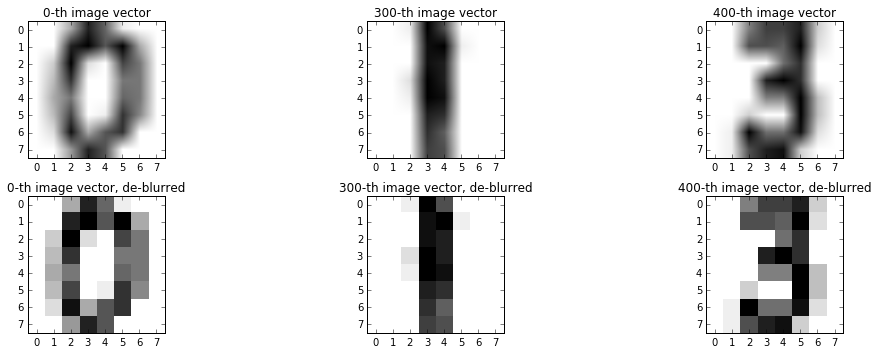Explain why PCA is a better choice for dimension reduction in this problem than step-wise variable selection

PCA finds the directions of maximum variability in the data. The features in this problem are the individual pixel values and as all pixels contribute to an image PCA is better choice for dimension reduction as the PCA components vectors are a linear combination of all the features instead of simply selecting a subset of the features. In addition, image arrays are high dimensional, and so step-wise variable selection would be computationally very inefficient (have to try very large number of combinations of the variables).

### Part 1(a). Reduce the data¶

Images data are typically high dimensional (the image vector has one feature for every pixel). Thus, to make working with image data more tractible, one might first apply a dimension reduction technique to the data.

• Explain why PCA is a better choice for dimension reduction in this problem than step-wise variable selection.
• Choose the smallest possible number of dimensions for PCA that still permits us to perform classification.

(Hint: how do we visually verify that subgroups in a dataset are easily classifiable?)

• Visualize and interpret the principal components. Interpret, also, the corresponding PCA varaiable values.
In :
#Let's project the data onto some random 2D planes
fig, ax = plt.subplots(1, 3, figsize=(15, 5))
alpha_var = 0.3

#Project onto axes: 1, 2
x_2d = x[:, [1, 2]]

ax.scatter(x_2d[y==0, 0], x_2d[y==0, 1], color='darkblue', alpha = alpha_var, label='0')
ax.scatter(x_2d[y==1, 0], x_2d[y==1, 1], color='darkred', alpha = alpha_var, label='1')
ax.scatter(x_2d[y==3, 0], x_2d[y==3, 1], color='darkgreen', alpha = alpha_var, label='3')

ax.set_xlabel('X_1')
ax.set_ylabel('X_2')
ax.set_title('Project of Data onto X_1 and X_2')
ax.legend()

#Project onto axes: 10, 20
x_2d = x[:, [10, 20]]

ax.scatter(x_2d[y==0, 0], x_2d[y==0, 1], color='darkblue', alpha = alpha_var, label='0')
ax.scatter(x_2d[y==1, 0], x_2d[y==1, 1], color='darkred', alpha = alpha_var, label='1')
ax.scatter(x_2d[y==3, 0], x_2d[y==3, 1], color='darkgreen', alpha = alpha_var, label='3')

ax.set_xlabel('X_10')
ax.set_ylabel('X_20')
ax.set_title('Project of Data onto X_1 and X_2')
ax.legend()

#Project onto axes: 30, 63
x_2d = x[:, [30, -1]]

ax.scatter(x_2d[y==0, 0], x_2d[y==0, 1], color='darkblue', alpha = alpha_var, label='0')
ax.scatter(x_2d[y==1, 0], x_2d[y==1, 1], color='darkred', alpha = alpha_var, label='1')
ax.scatter(x_2d[y==3, 0], x_2d[y==3, 1], color='darkgreen', alpha = alpha_var, label='3')

ax.set_xlabel('X_30')
ax.set_ylabel('X_63')
ax.set_title('Project of Data onto X_30 and X_63')
ax.legend()

plt.tight_layout()
plt.show()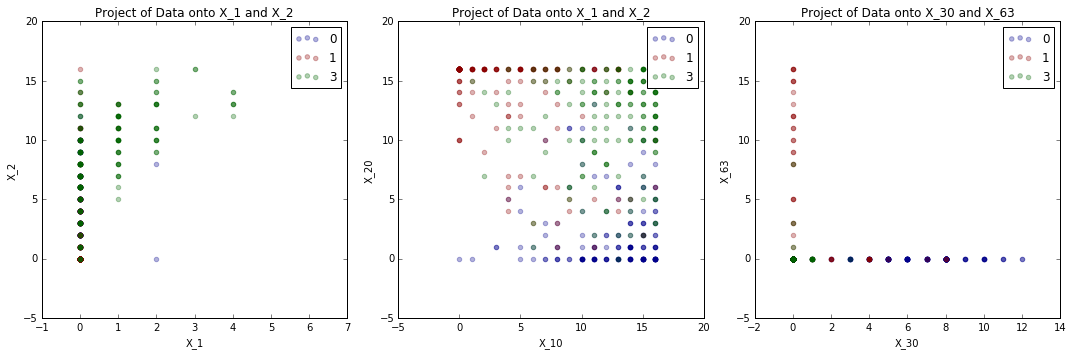Are these projections useful? That is do they reduce the data in a way that makes it possible for us to perform our task?

In :
#Let's project the data onto some random 2D planes
fig = plt.figure(figsize=(15, 5))
alpha_var = 0.3

#Project onto axes: 1, 2, 3
x_2d = x[:, [1, 2, 3]]

ax1 = fig.add_subplot(1, 3, 1,  projection='3d')

ax1.scatter(x_2d[y==0, 0], x_2d[y==0, 1], x_2d[y==0, 2], c='darkblue', color='darkblue', alpha = alpha_var, label='0')
ax1.scatter(x_2d[y==1, 0], x_2d[y==1, 1], x_2d[y==1, 2], c='darkred', color='darkred', alpha = alpha_var, label='1')
ax1.scatter(x_2d[y==3, 0], x_2d[y==3, 1], x_2d[y==3, 2], c='darkgreen', color='darkgreen', alpha = alpha_var, label='3')

ax1.set_xlabel('X_1')
ax1.set_ylabel('X_2')
ax1.set_zlabel('X_3')
ax1.set_title('Project of Data onto X_1, X_2, X_3')
ax1.legend(loc='lower left')

#Project onto axes: 10, 20, 30
x_2d = x[:, [10, 20, 30]]

ax2 = fig.add_subplot(1, 3, 2,  projection='3d')

ax2.scatter(x_2d[y==0, 0], x_2d[y==0, 1], x_2d[y==0, 2], c='darkblue', color='darkblue', alpha = alpha_var, label='0')
ax2.scatter(x_2d[y==1, 0], x_2d[y==1, 1], x_2d[y==1, 2], c='darkred', color='darkred', alpha = alpha_var, label='1')
ax2.scatter(x_2d[y==3, 0], x_2d[y==3, 1], x_2d[y==3, 2], c='darkgreen', color='darkgreen', alpha = alpha_var, label='3')

ax2.set_xlabel('X_10')
ax2.set_ylabel('X_20')
ax2.set_zlabel('X_30')
ax2.set_title('Project of Data onto X_10, X_20, X_30')
ax2.legend(loc='lower left')

#Project onto axes: 1, 50, 63
x_2d = x[:, [1, 50, 63]]

ax3 = fig.add_subplot(1, 3, 3,  projection='3d')

ax3.scatter(x_2d[y==0, 0], x_2d[y==0, 1], x_2d[y==0, 2], c='darkblue', color='darkblue', alpha = alpha_var, label='0')
ax3.scatter(x_2d[y==1, 0], x_2d[y==1, 1], x_2d[y==1, 2], c='darkred', color='darkred', alpha = alpha_var, label='1')
ax3.scatter(x_2d[y==3, 0], x_2d[y==3, 1], x_2d[y==3, 2], c='darkgreen', color='darkgreen', alpha = alpha_var, label='3')

ax3.set_xlabel('X_1')
ax3.set_ylabel('X_50')
ax3.set_zlabel('X_63')
ax3.set_title('Project of Data onto X_1, X_50, X_63')
ax3.legend(loc='lower left')

plt.tight_layout()
plt.show()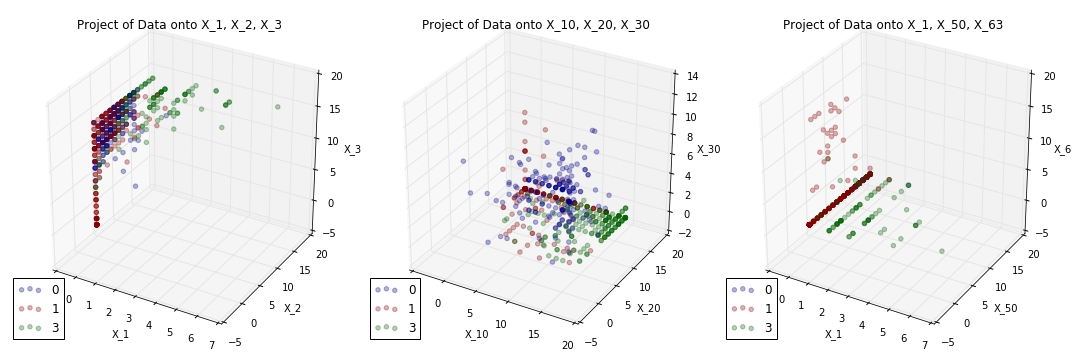Are these projections useful? That is do they reduce the data in a way that makes it possible for us to perform our task?

Let's now try reducing the number of dimensions in the data using PCA. Why is PCA a valid technique for dimension reduction? Why is PCA appropriate in the context of our task?

In :
#Apply PCA to data and get the top 3 axes of maximum variation
pca = PCA(n_components=3)
pca.fit(x)

#Project to the data onto the three axes
x_reduced = pca.transform(x)

#Visualized our reduced data
fig = plt.figure(figsize=(15, 5))
alpha_var = 0.5

ax1 = fig.add_subplot(1, 2, 1,  projection='3d')
ax1.scatter(x_reduced[y==0, 0], x_reduced[y==0, 1], x_reduced[y==0, 2], c='darkblue', color='darkblue', alpha = alpha_var, label='0')
ax1.scatter(x_reduced[y==1, 0], x_reduced[y==1, 1], x_reduced[y==1, 2], c='darkred', color='darkred', alpha = alpha_var, label='1')
ax1.scatter(x_reduced[y==3, 0], x_reduced[y==3, 1], x_reduced[y==3, 2], c='darkgreen', color='darkgreen', alpha = alpha_var, label='3')

ax1.set_xlabel('Component 1')
ax1.set_ylabel('Component 2')
ax1.set_zlabel('Component 3')
ax1.set_title('data projected onto the first 3 PCA components')
ax1.legend()

#Apply PCA to data and get the top 2 axes of maximum variation
pca = PCA(n_components=2)
pca.fit(x)

#Project to the data onto the three axes
x_reduced = pca.transform(x)

#Visualized our reduced data
ax2.scatter(x_reduced[y==0, 0], x_reduced[y==0, 1], c='darkblue', color='darkblue', alpha = alpha_var, label='0')
ax2.scatter(x_reduced[y==1, 0], x_reduced[y==1, 1], c='darkred', color='darkred', alpha = alpha_var, label='1')
ax2.scatter(x_reduced[y==3, 0], x_reduced[y==3, 1], c='darkgreen', color='darkgreen', alpha = alpha_var, label='3')

ax2.set_xlabel('Component 1')
ax2.set_ylabel('Component 2')
ax2.set_title('data projected onto the first 2 PCA components')
ax2.legend()

plt.tight_layout()
plt.show()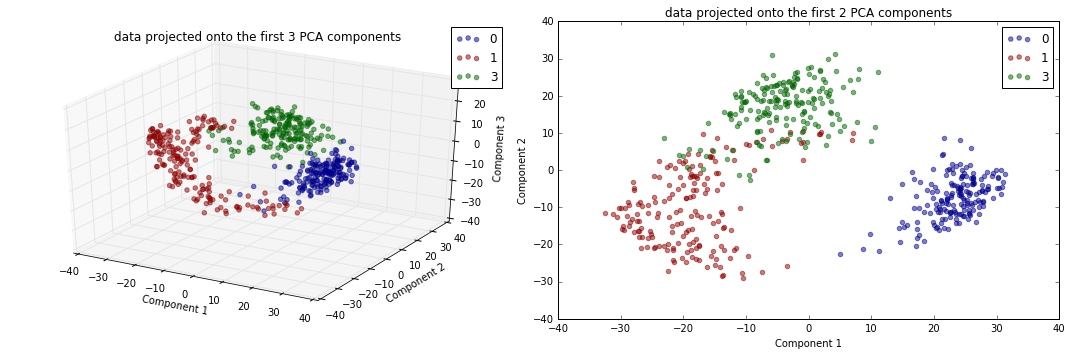Are the PCA dimension reductions any good? What does a "good" dimension reduction mean in the context of our task?

Can you interpret the components of the PCA in the context of our application? What is a component of the PCA (in terms of predictors) again?

In :
#Display the principal components of PCA as digital images
fig, ax = plt.subplots(2, 2, figsize=(10, 6))
# COMPONENT 1
ax[0, 0].imshow(pca.components_.reshape(8,8), cmap=plt.cm.gray_r)
ax[0, 0].set_title('Component 1')

# COMPONENT 2
ax[0, 1].imshow(pca.components_.reshape(8,8), cmap=plt.cm.gray_r)
ax[0, 1].set_title('Component 2')

# COMPONENT 1
ax[1, 0].imshow(pca.components_.reshape(8,8), cmap=plt.cm.gray_r, interpolation='nearest')
ax[1, 0].set_title('Component 1: de-blurred')

# COMPONENT 2
ax[1, 1].imshow(pca.components_.reshape(8,8), cmap=plt.cm.gray_r, interpolation='nearest')
ax[1, 1].set_title('Component 2: de-blurred')

plt.tight_layout()
plt.show()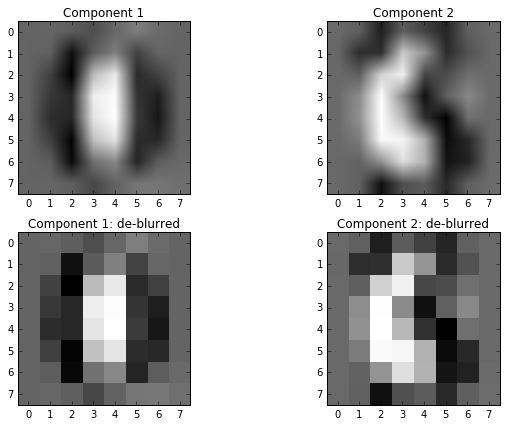### Part 1(b). Build a classifier¶

So far, we have only learned models that distinguishes between two classes. Develop and implement a simple and naive method of distinguishing between the three digits in our reduced dataset using binary classifiers.

In :
###Build a classifier to distinguish between 0 and 1

#Remove all instances of class 3
x_binary = x_reduced[y != 3, :]

#Remove all instances of class 3
y_binary = y[y != 3]

#Fit logistic regression model for 0 vs 1
logistic_01 = LogReg()
logistic_01.fit(x_binary, y_binary)

###Build a classifier to distinguish between 1 and 3

#Remove all instances of class 0
x_binary = x_reduced[y != 0, :]

#Remove all instances of class 0
y_binary = y[y != 0]

#Fit logistic regression model for 1 vs 3
logistic_13 = LogReg()
logistic_13.fit(x_binary, y_binary)

###Build a classifier to distinguish between 0 and 3

#Remove all instances of class 1
x_binary = x_reduced[y != 1, :]

#Remove all instances of class 1
y_binary = y[y != 1]

#Fit logistic regression model for 0 vs 3
logistic_03 = LogReg()
logistic_03.fit(x_binary, y_binary)

#Predict a label for our dataset using each binary classifier
y_pred_01 = logistic_01.predict(x_reduced)
y_pred_13 = logistic_13.predict(x_reduced)
y_pred_03 = logistic_03.predict(x_reduced)

#Now, for each image, we have THREE predictions!
#To make a final decision for each image, we just take a majority vote.
n = x_reduced.shape

y_votes[y_pred_01 == 0, 0] += 1
y_votes[y_pred_03 == 0, 0] += 1

y_votes[y_pred_01 == 1, 1] += 1
y_votes[y_pred_13 == 1, 1] += 1

y_votes[y_pred_03 == 3, 2] += 1
y_votes[y_pred_13 == 3, 2] += 1

#For each image, label it with the class that get the most votes

#Relabel class 2 as class 3
y_pred[y_pred == 2] = 3

#Accuracy of our predictions
print 'Accuracy of combined model:', np.mean(y == y_pred)

Accuracy of combined model: 0.935543278085


Is this accuracy meaningful? We've already seen cases where high R^2 values can be deeply misleading. Is an high accuracy rate mean we've learned a good classifier? Shouldn't there be a more intuitive way to assess the quality of our classifiers?

### Part 1(c). Build a better one¶

Asses the quality of your classifier.

• What is the fit (in terms of accuracy or R^2) of your model on the reduced dataset? Visually assess the quality of your classifier by plotting decision surfaces along with the data. Why is visualization of the decision surfaces useful? What does this visualization tell you that a numberical score (like accuracy or R^2) cannot?
• What are the draw backs of your approach to multi-class classification? What aspects of your method is contributing to these draw backs, i.e. why does it fail when it does?

(Hint: make use your analysis in the above; think about what happens when we have to classify 10 classes, 100 classes)

• Describe a possibly better alternative for fitting a multi-class model. Specifically address why you expect the alternative model to outperform your model.

(Hint: How does sklearn's Logistic regression module handle multiclass classification?).

In :
#--------  fit_and_plot_model
# A function to fit a binary LogReg model and visualize it
# Input:
#      model (LogReg model)
#      ax (axes object for plotting)
#      legend_label (legend label for the plot)

def plot_model(model, ax, legend_label, color):
#Get the coefficients from logistic regression model
coef = model.coef_
intercept = model.intercept_

#Find the max and min horizontal values of our data
x_0 = np.min(x_reduced[:, 0])
x_1 = np.max(x_reduced[:, 0])

#Plug int the max and min horizontal values of our data into the equation
#of the line defined by the coefficients
y_0 = (-intercept - coef * x_0) / coef
y_1 = (-intercept - coef * x_1) / coef

#Plot a line through the pair of points we found above
ax.plot([x_0, x_1], [y_0, y_1], label=legend_label, color=color)

fig, ax = plt.subplots(1, 1, figsize=(10, 6))
alpha_var = 0.5

#Scatter plot of our data
ax.scatter(x_reduced[y==0, 0], x_reduced[y==0, 1], color='darkblue', alpha = alpha_var, label='0')
ax.scatter(x_reduced[y==1, 0], x_reduced[y==1, 1], color='darkred', alpha = alpha_var, label='1')
ax.scatter(x_reduced[y==3, 0], x_reduced[y==3, 1], color='darkgreen', alpha = alpha_var, label='3')

#Plot decision boundaries for 0 vs 1
plot_model(logistic_01, ax, '0 vs 1', 'magenta')
#Plot decision boundaries for 1 vs 3
plot_model(logistic_13, ax, '1 vs 3', 'cyan')
#Plot decision boundaries for 0 vs 3
plot_model(logistic_03, ax, '0 vs 3', 'orange')

ax.set_xlabel('Component 1')
ax.set_ylabel('Component 2')
ax.set_xlim([np.min(x_reduced[:,0]), np.max(x_reduced[:,0])])
ax.set_ylim([np.min(x_reduced[:,1]), np.max(x_reduced[:,1])])
ax.set_title('our reduced data with all three decision boundaries (from binary classifiers)')
ax.legend()
plt.show()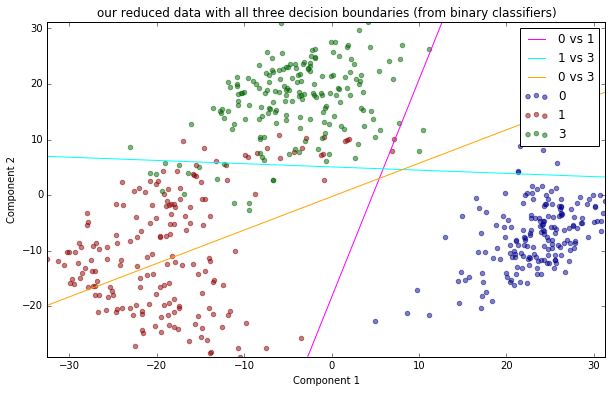## Problem 2. Sentiment Analysis¶

In this problem, you will explore how to predict the underlying emotional tone of textual data - this task is called sentiment analysis.

You will be using the dataset in the file dataset_2.txt. In this dataset, there are 1382 posts containing textual opinions about Ford automobiles, along with labels indicating whether the opinion expressed is positive or negative.

Given a new post about an automobile, your goal is to predict if the sentiment expressed in the new post is positive or negative. For this task you should implement a regularized logistic regression model.

Produce a report summarizing your solution to this problem:

• Your report should address all decisions you made in the "Data Science Process" (from Lectures #0, #1, #2):

a. Data collection & cleaning

b. Data exploration

c. Modeling

d. Analysis

e. Visualization and presentation

• Your report should be informative and accessible to a general audience with no assumed formal training in mathematics, statistics or computer science.
• The exposition in your report, not including code, visualization and output, should be at least three paragraphs in length (you are free to write more, but you're not required to).

Structure your presentation and exposition like a professional product that can be submitted to a client and or your supervisor at work.

## Introduction¶

The goal of this analysis is to build model in order to predict the emotional response based on textual data about Ford automobiles. That means predicting if the expressed sentiment is positive or negative.

## Functions (necessary for the following calculations)¶

In :
#--------  extract_words
# A function to extract words from a corpus
# requires
#      re
# Input:
#      corpus (corpus objec)
def extract_words(corpus):
import re

# Create array with all the sentences
whole_text = " ".join(corpus)

# Extract individual words
words = re.findall(r'\w+', whole_text.lower())
return words

#--------  count_words
# A function to count words
# requires:
#      pandas
#      collections
# Input:
#      words (word array)
#      number_of_words (number of most common words for the output)
def count_words(words, number_of_words):
common_words = pd.DataFrame(Counter(words).most_common(number_of_words), columns=["word", "frequency"])
return common_words

#--------  plot_common_words
# Plot histogram of the most common words
# requires:
#      matplotlib
# Input:
#      common_words (array of the words to plot)
def plot_common_words(common_words):

plt.figure(figsize=(18, 10))
plt.bar(common_words.index, common_words['frequency'].values, color = "darkblue", alpha = 0.7, edgecolor="white")
plt.xticks(common_words.index, common_words['word'].values, rotation=90)
plt.title('Plot I: 100 most common words')
plt.xlabel('Words'); plt.ylabel('Frequency')
plt.grid()
plt.show()


## 1: Importing Data¶

The data used containts 1'382 postings containing textual opinions about Ford automobiles, along with labels indicating whether the opinion expressed is positive or negative.

In :
# Load the data
print "The first 10 values of the dataset:"

The first 10 values of the dataset:

Out:
class text
0 Neg In 1992 we bought a new Taurus and we really ...
1 Neg The last business trip I drove to San Franci...
2 Neg My husband and I purchased a 1990 Ford F250 a...
3 Neg I feel I have a thorough opinion of this truc...
4 Neg AS a mother of 3 all of whom are still in ca...
5 Neg The Ford Winstar is a car that I would not re...
6 Neg We bought this van in 1999 after having been...
7 Neg I bought the Focus wagon for it s cargo space...
8 Neg You ve probably heard about the giant 2000 Fo...
9 Neg If you thought that the GMC Chevrolet Suburba...

## 2: Data exploration¶

The next step is exploring the data at hand. That means exploring the number of unique words in the corpus (entire set of reviews) and the counts of each word. In order to do that the paragraphs have to be transformed into a list containing the counts for each of the words, i.e., extracting the feature. This gives us a way of characterising the words present in each paragraph.

In :
# Create a corpus variable, i.e., all the articles in the dataset
corpus = data['text'].values

# Count classes
target_class = Counter(data['class'])

# Print the number of unique words
vectorizer_none = CountVectorizer()
total_words = len(vectorizer_none.fit(corpus).get_feature_names())
print 'Total number of unique words in the corpus: ', total_words
print 'Total number of negativ values: ', target_class.values()
print 'Total number of positive values: ', target_class.values()

Total number of unique words in the corpus:  16730
Total number of negativ values:  691
Total number of positive values:  691


Analysing the data shows that there are 16'730 unique words in the corpus. Furthermore, the distribution of the phares is equaly distributed (50/50), i.e., 691 are postive and 691 are negative.

In :
# The corpus is composed of all the articles in the dataset.
words = extract_words(corpus)
common_words = count_words(words, len(words))
plot_common_words(common_words[0:99])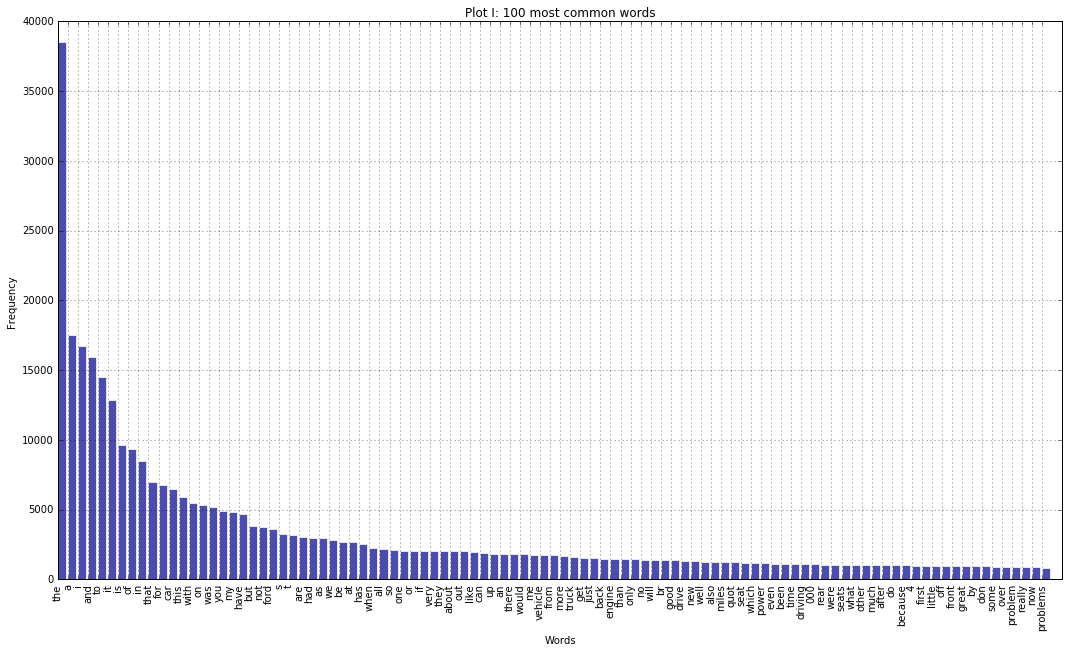Visualizing the most frequent words shows, that there are filler words occuring in the dataframe. Those filler or stopwords such as 'the', 'a' etc. are beeing remove as they have no relationship with the sentiment expressed, i.e., they do no contribute to the positivity/negativity of an article. Furthermore, words that occur fewer than 30 time across the entire dataset are also remove. This number is chosen as it can be shown that after a occurence of n>30 the mean distrubtion start approximating a normal distribution. Having words occuring fewer than 30 times either could lead to spurios relationsships or are in the case of n=1 nonsensical.

## 3: Cleaning the data¶

In :
# Extract the words
vec_common_words = common_words['word'].values

## Select manually filler words (among the most common words)
filler_words = ['the', 'a', 'i', 'and', 'to', 'it', 'is', 'of', 'in', 'that', 'for', 'this', 'with', 'on', 'was', 'you', 'my',
'have', 'but', 'not', 'ford', 's', 't', 'are', 'had', 'as', 'we', 'be', 'at', 'has', 'when', 'all', 'so', 'one', 'or',
'if', 'very', 'they', 'about', 'out', 'like', 'up', 'an', 'there', 'would', 'me', 'vehicle', 'from', 'more',
'truck', 'get', 'just', 'back', 'engine', 'than', 'will', 'br', 'drive', 'also',
'miles', 'quot', 'seat', 'which', 'power', 'even', 'been', 'time', 'driving', '000', 'rear', 'were', 'seats', 'what',
'other', 'much', 'after', 'do', 'because', '4', 'first', 'off', 'front', 'by', 'don', 'some', 'over',
'now', 'cars', 'its', 'any', 'two']

# Select words where there are fewer than 30 occurences --> danger of overfitting
small_freq_words = common_words['word'][common_words['frequency'] <= 30].values
filler_words = filler_words
stop_arr = filler_words + list(small_freq_words)
#print "Words too keep: ", [w for w in vec_common_words if w not in stop_arr]

In :
# Create dummy vector
dummy_y = pd.get_dummies(data['class'])
df = pd.concat([dummy_y, data['text']], axis=1)

In :
## Removing filler words words
vectorizer = CountVectorizer(stop_words=stop_arr)

# Create a feature vector for each article
vector_transform = vectorizer.fit(corpus)
x = vectorizer.transform(corpus)

# Feature extraction (inverse document frequencey scaling)
transformer_object = TfidfTransformer()
x_object = transformer_object.fit_transform(x)

# Extract y and x matrices
y = df['Pos'].values
x = x_object.toarray()

print 'Shape of transformed article data: ', x.shape
print 'Number of features: ',  len(vectorizer.get_feature_names())

# Source: http://stackoverflow.com/questions/39898477/tf-idf-score-from-tfidftransformer-in-sklearn-on-same-word-in-two-sentences-with

Shape of transformed article data:  (1382L, 1665L)
Number of features:  1665


Having clean the data and prepared it for the modelling part the number of features is reduced to 1'665 features. The data can now be used to build a statistical model in order to predict the sentiment.

## 4. Modelling¶

As described above, the transformed data can in the following step be used to fit a a statistical model (logistic regression) on the data. This model can then be usd to predict the sentiment of an article given it's feature vector i.e the occuring words in a paragraph/review.

Before that can be done the data has to be split into two sets - a training and a testing set. The training set is used to build the model while the test set is used determine the accuracy of the model. This is done in order to have the ability to check if the build model is able to perform accuratly on data not used to build the model.

In :
# Create testing and training set (70% Train, 30% Test)
np.random.seed(123) # Set random seed
x_train, x_test, y_train, y_test = train_test_split(x, y, test_size=0.3)


As the data is with 1'665 features high dimensional, i.e., care has to be taken that the model doesn't overfit the data. Overfitting means that the model fits too closely to the available data and does not generate useful predictions for new paragraphs.

There are 3 measures taken in order to prevent this issues. The first is to use a technique called regularisation, the second crossvalidation and the third the already described step of using test and training data. The regularisation bit introduces a penality for complexity while a 10 fold cross validation is used to choose the best value and best model.

In :
# Create logistic regression object
# c = 1/lamda --> Tuning parameter for regularization --> big c --> no regularization
logitm_cv = LogRegCV(Cs=100, cv=10, penalty="l2")
logitm_cv.fit(x_train, y_train)

Out:
LogisticRegressionCV(Cs=100, class_weight=None, cv=10, dual=False,
fit_intercept=True, intercept_scaling=1.0, max_iter=100,
multi_class='ovr', n_jobs=1, penalty='l2', random_state=None,
refit=True, scoring=None, solver='lbfgs', tol=0.0001, verbose=0)
In :
# Calculate scores
cv_scores = logitm_cv.scores_[1.0]
mean_score = np.mean(cv_scores, axis=0)

In :
# Plotting
plt.figure(figsize=(18, 10))
plt.semilogx(logitm_cv.Cs_, mean_score, color='darkblue', linewidth=3)
plt.title('Plot II: CV Parameters')
plt.xlabel('Regularisation parameter'); plt.ylabel('Mean score')
plt.grid()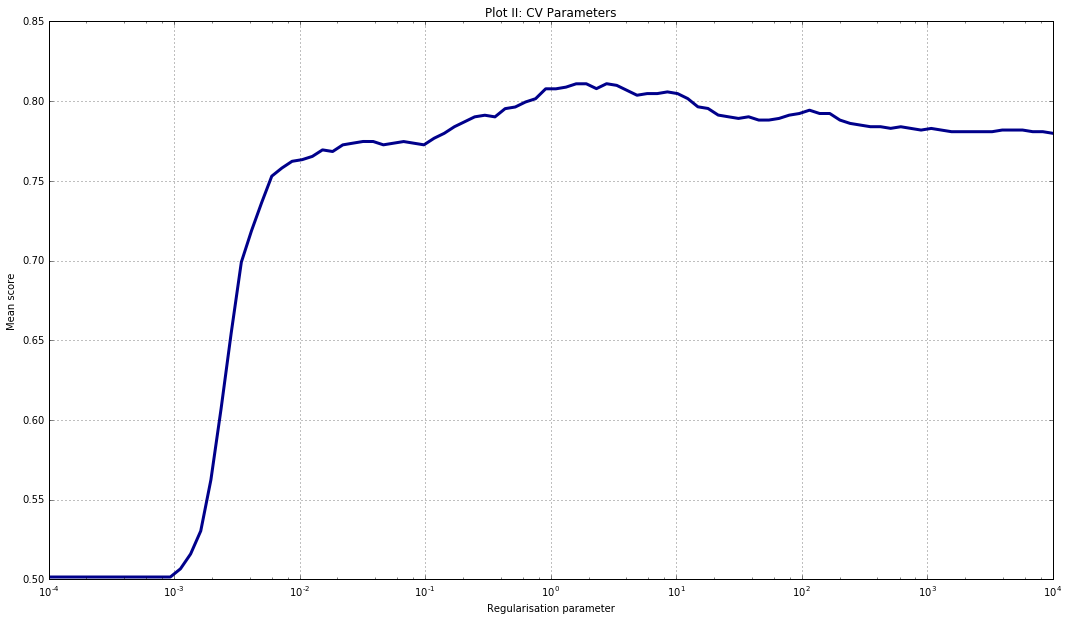The above cross validation plot shows, that for very small values of the regularisation parameter, the penalty leads to a random, i.e., 50/50 model. That means, that the model performes like chance. With a bigger regularisation parameter the penalty goes lower and the score gets better up to a point and then goes down again. This effect occurs because of overfitting.

In :
# Find the optimal c-value (regularisation parameter)
print 'Optimal c-value: ', logitm_cv.C_
print 'Mean score: ', max(mean_score)

Optimal c-value:  2.78255940221
Mean score:  0.810965442878


The optimal c-value is around 2.78 while that gives a mean score of 0.81 on the training data. Those values can now be used to build the logistic regression model.

In :
# Build logistic model with the above c-value
logitm_model = LogReg(C = logitm_cv.C_, penalty = "l2")
logitm_model.fit(x_train, y_train)

Out:
LogisticRegression(C=2.7825594022071258, class_weight=None, dual=False,
fit_intercept=True, intercept_scaling=1, max_iter=100,
multi_class='ovr', n_jobs=1, penalty='l2', random_state=None,
solver='liblinear', tol=0.0001, verbose=0, warm_start=False)

## 5. Analysis:¶

After the model parameters have been determined, the next step is to make predictions on the testing set.

In :
# Predicting on the test set
y_pred = logitm_model.predict(x_test)

# Calculate the false negative and false positive scores
false_negative_indices = [i for i, x in enumerate(y_test) if ((y_pred[i] == 0) and (y_test[i] == 1))]
false_positive_indices = [i for i, x in enumerate(y_test) if ((y_pred[i] == 1) and (y_test[i] == 0))]

# Printing the output
print 'Accuracy of the model', logitm_model.score(x_test, y_test)
print 'Number of false positives', len(false_positive_indices)
print 'Number of false negatives', len(false_negative_indices)

---------------------------------------------------------------------------
NameError                                 Traceback (most recent call last)
<ipython-input-1-e35fe74a1c69> in <module>()
1 # Predicting on the test set
----> 2 y_pred = logitm_model.predict(x_test)
3
4 # Calculate the false negative and false positive scores
5 false_negative_indices = [i for i, x in enumerate(y_test) if ((y_pred[i] == 0) and (y_test[i] == 1))]

NameError: name 'logitm_model' is not defined

The accuracy of the model can be estimated trough the percentage of correct predictions made on the test set i.e. the number of reviews for which the predicted sentiment matches the given sentiment. In addition, the number of false postives (model predicts postive, but actually negative) and false negatives (model predicts negative but actually postive) can be investigated.

The accuracy of the trained model is around 80%, i.e. the model predicts, based on the text in the review, in 80 out of 100 cases the correct sentiment. In the set of false predictions (positives and negatives) the rate is equal indicating that the model doesn't have a bias towards making postive or negative predictions.

The next step is to interpret the model by looking at the coefficients. There is one coefficient for every occuring word. The model has high numbers for words which contribute to making a review positive, while having low numbers for words associated with negative reviews.

In :
# Negative words
sorted_coeff_indices = np.argsort(logitm_model.coef_)
x_features = np.array(vectorizer.get_feature_names())
negative_words = x_features[sorted_coeff_indices[0:20]]

# Positive words
positive_words = x_features[sorted_coeff_indices[-20:]]

# Combine
df_pos_neg = pd.concat([pd.Series(positive_words), pd.Series(negative_words)], axis=1)
df_pos_neg.columns = ["Positive words", "Negative words"]

# Print
df_pos_neg

Out:
Positive words Negative words
0 wheel transmission
1 excellent broke
2 mileage uncomfortable
3 regular cost
4 trips weeks
5 cargo he
6 146s problems
7 little repair
8 snow worst
9 am then
10 escort did
12 ve toyota
13 perfect fixed
14 highly months
15 ride started
17 comfortable rental
18 love 3rd
19 great these

The top 20 positive and top 20 negative words are shown above. From the positive words it can be seen that words like 'excellent', 'perfect', 'comfortable', 'love', etc. are correlated with a positive review while words like 'broke', 'uncomfortable', 'cost', 'bad', etc. are correlated with a negative review.

## 6. Visualisation:¶

As a next step the confidence score for each article can be calculated and visualized. A histogram is a good form to do this. A confidence score smaller than 0 means, that the class = negative is predicted. On the other side a score smaller than zero leads to a positive prediction.

In :
# Confidence scores for negatives
y_test_neg_prob = logitm_model.decision_function(x_test[y_test==0, :])

# Confidence scores for positives
y_test_pos_prob = logitm_model.decision_function(x_test[y_test==1,:])

# Normal mu and se
(mu_pos, sigma_pos) = norm.fit(y_test_pos_prob)
(mu_neg, sigma_neg) = norm.fit(y_test_neg_prob)

In :
# Plotting
bins = np.linspace(-4, 4, 50) # Create bins
plt.figure(figsize=(18, 10))
plt.hist(y_test_pos_prob, bins, alpha=0.7, color='darkblue', edgecolor="white", label='Postive articles')
plt.hist(y_test_neg_prob, bins, alpha=0.7, color='darkred', edgecolor="white", label='Negative articles')
plt.axvline(x=0, linewidth=8, color='black', alpha = 0.7)
plt.title('Plot II: Histogram of the confidence scores')
plt.xlabel('Confidence score')
plt.ylabel('Count of the number of words')
plt.legend(loc='best')
plt.grid()
plt.show()